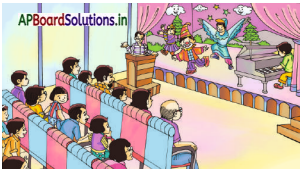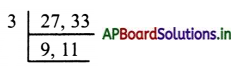# AP Board 5th Class Maths Solutions 5th Lesson Multiples and Factors

Andhra Pradesh AP Board 5th Class Maths Solutions 5th Lesson Multiples and Factors World Textbook Exercise Questions and Answers.

## AP State Syllabus 5th Class Maths Solutions Chapter 5 Multiples and Factors

Question 1.
Observe which numbers are not divisible?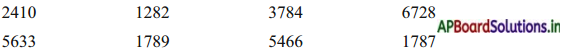Answer: Which of the above numbers are exactly divisible by 2?
2410, 1282, 3784, 6728, 5466

b. Observe the units place of the numbers which are divisible by 2.
0, 2, 4, 6, 8.

c. Are all these numbers even numbers ? Yes / no. (Yes)
So a number is divisible by 2, if the digit at its one place is either 0/2/4/6/8.Do these: (TextBook Page No.62)

Question 1.
Circle the following numbers which are divisible by 2.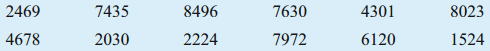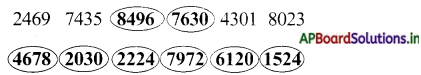Question 2.
Write any 5, four-digit numbers which arc divisible by 2 ?
25680, 45,622, 78,964, 87,766 and 97.678

Exercise 1:

Question 1.
Find the numbers which are divisible by 2. Write the reason for the numbers which are not divisible?
a) 3458
b)56745
c) 3850
d) 6736
e) 6733
f) 3394
3458, 3850, 6736 and 3394 are divisible by 2. Remaining numbers are not divisible.
Reason : The digits of the numbers of ones places is 3 and 5.Question 2.
Find the numbers which are divisible by 5 and 19. Write the reason for the numbers which are not divisible ?
a) 3568
b) 3540
c) 6585
d) 7550
e) 4235
f) 7200
g) 7865
h) 5880
i) 4440
j) 8198
k) 8645
b, d, f, h, j are divisible by both 5 and 10. Remaining numbers are not divisible by 5 and 10.
Reason : The numbers which have n’t zero at their ones place.

Question 3.
The numbers below are divisible by 5. Fill in the blanks with suitable digit.
a) 786_
786_ (0/5)

b)560_
560_(0/5)

c)785_
785_(0/5)

d) 555_
555_ (0/5)

e) 586_
586(0/5)

f) 786_
786_(0/5)

g) 584_
584_(0/5)

h) 100_
100_(0/5)

Question 4.
Write any 5 numbers which are exactly divisible by 2 and 5.
2540, 62570, 250, 367280 and 764520.

Question 5.
Write any 5 numbers which are exactly divisible by 2,5 and 10 ?
86540, 79980, 89960, 45570 and 76540.Do these: (TextBook Page No.65)

Question 1.
Circle the number which is exactly divisible by 3 and 9 and write correct reason.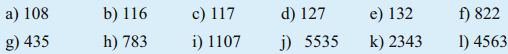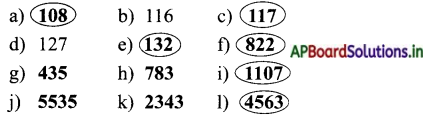Reason : If the digital root of the given number is 9, then the number is exactly divisible by 3 and 9.

Question 2.
Write any 5 numbers which are exactly divisible by 3 and 9?
1350, 1476, 0342, 1539 and 1629.

Do these: (TextBook Page No.66)

Question 1.
Circle the numbers which are divisible by 4. Give the reason, if is not divisible by 4.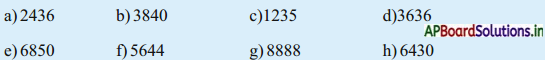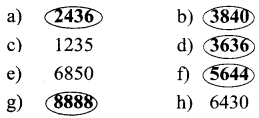a, b, d, f and g are divisible by 4. Remaining numbers last two digits is not divisible by 4. So they are not divisible by 4.

Question 2.
Write the missing number in the blank to make the number exactly divisible by 4.
a) 323_
323_ (2 / 6)

b) 304_
304_ (0)

c) 58_6
58_ 6 (1/3)

d) 53_ _
53_ _ (04 / 0812 / 16 / 20 / 24 / 28 / 36/ 40)

e) 65_ _
65_ _
(04 / 08 / 12 / 16 / 20 / 24 / 28/ 36 / 40).Do these (Textbook Page No. 67)

Question 1.
Check whether the following numbers are divisible by 6 or not.
1) 210
2) 162
3) 625
4) 120
5) 156
210, 162, 120 and 156 are divisible by ’6’.

Question 2.
Change the digits of the following numbers to make them divisible by 6.
1) 543
2) 231
3) 5463
4) 1002
5) 4815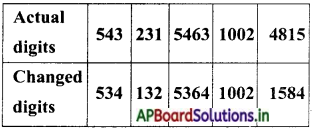Do these: (TextBook Page No.68)

Question 1.
Find the following numbers which are divisible by 8.
a) 2456
b) 3971
c) 824
d) 923
e) 2780
f) 93624
g) 76104
a) In 2456, 456 is divisible by 8. So, 2456 is divisible by 8.
b) In 3971, 971 is not divisible by 8. So, 3971 is not divisible by 8.
c) 824 is divisible by 8.
d) 923 is not divisible by 8.
e) In 2780, 780 is not divisible by 8. So, 2780 is not divisible by 8.
f) In 93624, 624 is divisible by 8. So, 93624 is divisible by 8.
g) In 76104, 104 is divisible by 8. So, 76104 is divisible by 8.Exercise 2:

Question 1.
Circle the following numbers which are divisible by 2 (by using divisibility rule).
3624 3549 7864 8420 8500 8646 5007 7788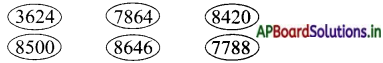Question 2.
Find out which of the following numbers are divisible by 6.
1276 43218 71218 71826 4734 3743
(i) Given number = 1276
Ones place is 6 that is even.
1276 is divisible by 2.
Digital root of 1276 is 1 + 2 + 7 + 6 = 16
16 is not divisible by 3.
So, 1276 is not divisible by 6.

(ii) Given number = 43218
Ones place is 8 that is even.
43218 is divisible by 2.
Digital root of 43218 is 4 + 3 + 2 + 1 + 8 = 18
18 is divisible by 3.
So, 43218 is divisible by ‘6’.

(iii) Given number = 71218
Ones place is 8 that is even.
71218 is divisible by ‘2’
Digital root of 71218 is
7 + 1 + 2 + 1 + 8 = 19
19 is not divisible by ‘3’
So, 71218 is divisible by ‘6’.

(iv) Given number = 71826
Ones place is ‘6’ that is even.
71826 is divisible by ‘2’
Digital root of 71826 is 7 + 1 + 8 + 2 + 6 = 24
24 is divisible by ‘3’
So, 71826 is divisible by’6′.

(v) Given number = 4734
Ones place is ‘4’ that is even.
4734 is divisible by ‘2’
Digital root of 4734 is 4 + 7 + 3 + 4 = 18
18 is divisible by’3′
So, 4734 is divisible by ‘6’.

(vi) Given number = 3743
Ones place is ‘3’ that is odd.
3743 is not divisible by ‘2’
So, 3743 is not divisible by ‘6’.Question 3.
The number 50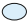9 is exactly divisible by 9. Fill thewith the correct number.
Given number 5019 is exactly divisible by 9.
So, digital root is 5 + 0 + ? + 1 + 9 = 15 + ?
= 15 + 3 = 18 = 1 + 8 = 9
∴ 9 is divisible by ‘9’.

Question 4.
The number 4468 is exactly divisible by 6. Fill thewith the correct number.
Given number 4468 is exactly divisible by 6. So, digital roots is 4 ++ 4 + 6 + 8 = 22 + 2 = 24
24 is divisible by 3.
So, 4 2 468 is divisible by 6.

Question 5.
Fill the blanks with suitable digits. So that it can be divisible by 2 and 10.
678_ 588_ 388_ 222_
364_ 786_ 666_ 788_
Given numbers are divisible by ’10’ they have ‘0’ in its units place and that number also divisible by ‘2’.
6780 5880 3880 2220
3640 7860 6660 7880

Question 6.
Find the numbers which are divisible by 4 and 8.
2104 726352 1800 32256 52248 25608
Observe the last two digits of the given numbers 2104, 726352, 1800, 32256, 52248 and 25608.
There are 04, 52, 00, 56, 48 and 08 at the end of the numbers.
Hence all these numbers are multiples of 4.
So, 2104, 726352, 1800, 32256, 52248 and 25608 are divisible by 4.

Divisible by 8 :
i) Given number 2104
Last three digits of given 104 is divisible by 8.
So, 2104 is divisible by 8.

ii) Given number 726352
Last three digits of given 352 is di-visible by 8.
So, 726352 is divisible by 8.

iii) Given number 1800
Last three digits of given 800 is divisible by 8.
So, 1800 is divisible by 8.

iv) Given number 32256
Last three digits of given 256 is divisible by 8.
So, 32256 is divisible by 8.

v) Given number 52248
Last three digits of given 248 is divisible by 8.
So, 52248 is divisible by 8.Question 7.
Try whether the numbers are divisible by 2, 3, 4, 5, 6, 8, 9 and 10.
a) 333
b) 128
c) 225
d) 7535
e) 8289
f) 99483
g) 67704
h) 67713
i) 9410
j) 67722
k) 20704
l) 35932
m) 85446
n) 90990
o) 18540
a) Given number is 333
Digital root of 333 is 3 + 3 + 3 = 9
Hence it is multiple of 3 and 9.
So, 333 is divisible by 3 and 9.

b) Given number is 128.
Ones place is 8 that is even.
128 is divisible by 2.
Last two digits of the given number is 28 is multiple of 4.
So, 128 is divisible by 4.
128 ÷ 8 = 16.
So, 128 is divisible by 8
Hence 128 is divisible by 2, 4 and 8.

c) Given number is 225
Ones place is ‘5’, so it is divisible by 5.

d) Given number is 7535
Ones place is 5, so, it is divisible by 5.e) Given number is 8289.
Digital root of 8289 is 8 + 2 + 8 + 9 = 27 = 2 + 7 = 9
9 is multiple of 3 and 9.
So, 8289 is divisible by 3 and 9.

f) Given number is 99483.
Digital root of 99483 is 9 + 9 + 4 + 8 + 3 = 33 = 3 + 3 = 6
6 is multiple of 3, so, 99483 is divisible by 3.

g) Given number is 67704
Ones place is 4 that is even.
67704 is divisible by 2.
Digital root of 67704 is 6 + 7 + 7 + 0 + 4 = 24 = 2 + 4 = 6.
6 is multiple of 3, so, 67704 is divisible by 3.
The number is divisible by 2 and 3, so, 67704 is divisible by 6.
Last two digits of the number is 04 is divisible by 4. So, 67704 is divisible by 4.
Last three digits of the number is 704 is divisible by 8.
So, 67704 is divisible by 8.
∴ 67704 is divisible 2, 3,4, 6 and 8.

h) Given number is 67713
Digital root of 67713 is 6 + 7 + 7 + 1 + 3 = 24 = 2 + 4 = 6
6 is multiple of 3.
So, 67713 is divisible of 3.

i) Given number is 9410
Last digit of given number is ‘0’ that is even.
So, 9410 is divisible by 2, 5 and 10.

j) Given number is 67722
Ones place is 2 that is even.
67722 is divisible by 2.
Digital root of 67722 is 6 + 7 + 7 + 2 + 2 = 24 = 2 + 4 = 6
‘6’ is divisible by 3.
So, 67722 is divisible by 6.

k) Given number is 20704.
Ones place is 4 that is even.
20704 is divisible by 2.
Last two digits of the number is 04 is divisible by 4.
So, 20704 is divisible by 4.
Last three digits of the number is 704 is divisible by 8. So, 20704 is divisible by 8.
∴ 20704 is divisible 2, 4 and 8

l) Given number is 35932
Ones place is 2 that is even.
35932 is divisible by 2.
Last two digits of the number is 32
32 is multiple of 4.
So, 35932 is divisible by 4.
∴ 35932 is divisible 2 and 4

m) Given number is 85446
Ones place is 6 that is even.
85446 is divisible by 2.
Digital root of 85446 is 8 + 5 + 4 + 4 + 6 = 27 = 2 + 7 = 9
∴ ‘9’ is multiple of 3 and 9.
So, 85446 is divisible by 3 and 9.
∴ 85446 is divisible by 2,3 and 9.

n) Given number is 90990
Last digit is ‘0’.
So, it is divisible by 2,5 and 10.

o) Given number is 18540 Last digit is ‘0’.
So it is divisible by 2, 5 and 10.Question 8.
Find the missing digit that would make each number divisible by the given number.
a) 395 _ by 10
395 0

b)24305 _ by 9
24305 4

c) 69839 _ by 3 and 9
698391

d) 271 _ 8 by 6
2710 8

e) 20710 _
20710_

f) 5027 _ 5 by 3 and 5
50273 5

g) 145 _ 2 by 8
14512

h) 92048 _ by 2
92048 _ (0/2/4/6/8)

i) 23405 _ by 5
(0/5)

Answer: For eiven ‘e’ problem divisible by is not given. So, the problem is not solved.Question 9.
Find the smallest number that is to be added to 289279, so that it can be divisible by 8.
Given number is 289279.
Last three digits of given number is 279.
279 is not divisible by 8, but 280 is divisible by 8.
289279 is added by 1 it is divisible by 8.

Do these: (TextBook Page No. 69 & 70)

Question 1.
Write first ten multiples of the following.
a) 3 b) 5 c) 8 d) 9 e) 10
a) Ten multiples of 3 = 3, 6, 9, 12, 15, 18, 21, 24, 27, 30
b) Ten multiples of 5 = 5, 10, 15, 20, 25, 30, 35, 40, 45, 50
c) Ten multiples of 8 = 8, 16, 24, 32, 40, 48, 56, 64, 72, 80
d) Ten multiples of 9 = 9, 18, 27, 36, 45, 54, 63, 72, 81, 90
e) Ten multiples of 10 = 10, 20, 30, 40, 50, 60, 70, 80, 90, 100

Question 2.
Find out the multiples of 2, 3, 5 from 1 to 20. Write separately.
First 1 to 20 multiples of 2 = 2, 4, 6, 8, 10, 12, 14, 16, 18, 20
First 1 to 20 multiples of 3 = 3, 6, 9, 12, 15, 18, 21, 24, 27, 30
First 1 to 20 multiples of 5 = 5, 10, 15,20

Question 3.
Write down the first 10 multiples of 7.
First 10 multiples of 7 is = 7, 14, 21, 28, 35, 42, 49, 56, 63, 70.

Question 4.
Find out the multiples of 7, 8, 10 from the following numbers and write separately.
20, 14, 45, 24, 32, 35, 90, 8, 7, 10, 441, 385
Multiples of 7 from given = 7, 14, 35, 385, 441
Multiples of 8 from given = 8, 24, 32
Multiples of 10 from given = 10, 20, 90

Question 5.
Find out the numbers which are not the multiples of 3.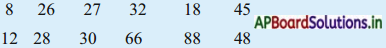8 26 32 28 88 48

Question 6.
Write the odd multiples of 9 less than hundred.
9, 27, 45, 63, 81, 99.Do these: (TextBook Page No.71)

Question 1.
Write first 10 multiples of the following numbers and list the com-mon multiples.
a) 2 and 4
b) 4 and 12
c) 6 and 8
d) 5 and 10
a) Multiples of 2 : 2, 4, 6, 8, 10, 12, 14, 16, 18, 20.
Multiples of 4 : 4, 8, 12, 16, 20, 24, 28, 32, 36, 40
Common multiples of 2 and 4 : 4, 8, 12, 16, 20

b) Multiples of 4 : 4, 8, 12, 16, 20, 24, 28, 32, 36, 40
Multiples of 12 : 12, 24, 36, 48, 60, 72, 84, 96, 108, 120
Common multiples of 4 and 12 : 12, 24, 36

c) Multiples of 6 : 6, 12, 18, 24, 30, 36, 42, 48, 54, 60
Multiples of 8 : 8, 16, 24, 32, 40, 48, 56, 64, 72, 80
Common multiples of 6 and 8 : 24, 48

d) Multiples of 5 : 5, 10, 15, 20, 25, 30, 35, 40, 45, 50
Multiples of 10 : 10, 20, 30, 40, 50, 60, 70, 80, 90, 100
Common multiples of 5 and 10 : 10, 20, 30, 40, 50.Do this: (TextBook Page No. 72)

Find the LCM for the following sets of numbers.
Multiples of 12 : 12, 24, 36, 48, 60, 72, 84, 96, 108, 120, …………..
Multiples of 15: 15, 30, 45, 60, 75, 90, 105, 120, 135, 150, …………
Common multiples of 12 and 15 = 60, 120
Least common multiple of 12 and 15 = 60.

2) Multiples of 16: 16, 32, 48, 64, 80, 96, 112, 128, 144, 160
Multiples of 20 : 20, 40, 60, 80, 100, 120, 140, 160, 180, 200, ………..
Common multiples of 16 and 20 = 80, 160.
Least common multiple of 16 and 20 = 80

3) Multiples of 8:
8, 16, 24, 32, 40, 48, 56, 64, 72, 80, ……….., 120, ………..
Multiples of 12: 12, 24, 36, 48, 60, 72, 84, 96, 108, 120, …………
Multiples of 20: 20, 40, 60, 80, 100, 120, 140, 160, 180, 200, …………
Least common multiple of 16 and 20 = 80
Common multiples of 8, 12 and 20 = 120
Least common multiple of 8, 12 and 20 = 120

4) Multiples of 15 : 15, 30, 45, 60, 75, 90, 105, 120, 135, 150 ……….
Multiples of 20 : 20, 40, 60, 80, 100, 120, 140, 160, 180, 200
Common multiples of 15 and 20 = 60, 120
Least common multiple of 15 and 20 = 60

5) Multiples of 6 : 6, 12, 18, 24, 30, 36, 42, 48, 54, 60, …………
Multiples of 9: 9, 18, 27, 36, 45, 54, 63, 72, 81, 90, ………..
Multiples of 12 : 12, 24, 36, 48, 60, 72, 84, 96, 108, 120
Common multiples of 6, 9 and 12 = 36
Least common multiple of 6, 9 and 12 = 36.Try this: (TextBook Page No. 72)

Find the LCM for the following pairs of numbers. What do you ob-serve.
1) 15, 30
2) 4, 16
3) 5, 15
4) 6, 18
1) Multiples of 15 : 15, 30, 45.60. 75.90, 105, 120, 135,150
Multiples of 30 : 30,60, 90. 120, 150, 180, 210, 240, 270, 300, …………
Common multiples of 15 and 30
30, 60, 90, 120, 150, ……………..
Least common multiples of 15 and 30 = 30

2) Multiples of 4 :
4, 8, 12, 16, 20, 24, 28, 32, 36, 40, …………
Multiples of 16 : 16, 32, 48, 64, 80, 96, 112, 128, 144, 160, …………..
Common multiples of 4 and 16 = 16, 32, 48 …………
LCM of 4 and 16 = 16

3) Multiples of 5 : 5, 10, 15, 20, 25, 30, 35, 40, 45, 50, 55, 60, …………..
Multiples of 15 : 15, 30, 45, 60, 75, 90, 105, 120, 135, 150, ………….
Common multiples of 5 and 15 = 15, 30, 45, 60
LCM of 5 and 15 = 15

4) Multiples of 6 :
6, 12, 18,24, 30, 36,42,48, 54, 60, ……………
Multiples of 18 :
18, 36, 54, 72,90, 108, 126, 144, 162, 180, ……….
Common multiples of 6 and 18 = 18, 36, 54
LCM of 6 and 18 = 18
Observation :
In a given pair of numbers, if one of them is multiple of other number than the biggest number is LCM of the number.Do these: (TextBook Page No.75)

Question 1.
Find all the factors of the following numbers.
a) 21
b) 38
c) 72
d) 96
a) 21 = 1 × 21 = 3 × 7 = 7 × 3 = 21 × 1
Thus, all the factors of 21 are : 1, 3, 7 and 21.

b) 38 = 1 × 38
= 2 × 19
Thus, all the factors of 38 are : 1, 2, 19 and 38.

c) 72 = 1 × 72
= 2 × 36
= 3 × 24
= 4 × 18
= 6 × 12
= 8 × 9
Thus, all the factors of 72 are : 1, 2, 3, 4, 6, 8, 9, 12, 18, 24, 36 and 72.

d) 96 = 1 × 96
= 2 × 48
= 3 × 32
= 4 × 24
= 6 × 16
= 8 × 12
Thus, all the factors of 96 are : 1, 2, 3, 4, 6, 8, 12, 16, 24, 32, 48 and 96.Question 2.
Find out whether the first number is a factor of the second number.
a) 14; 322
b) 26; 832
c) 35; 425
d) 56; 3500
e) 8; 48
f) 14; 37
g) 15; 75
h) 12; 72
a) Yes, 14 is a factor of 322.
b) Yes, 26 is a factor of 832.
c) No, 35 is not a factor of 425.
d) No, 56 is not a factor of 3500.
e) Yes, 8 is a factor of 48.
0 No, 14 is not a factor of 37.
g) Yes, 15 is a factor of 75.
h) Yes, 12 is a factor of 75.

Question 3.
What are the factors of 66 ?
Factors of 66 = 1 × 66
= 2 × 33
= 3 × 22
= 6 × 11
Factors are 1, 2, 3, 6, 11, 22, 33 and 66.Question 4.
Write all the even factors of 64.
Factors of 64 = 1 × 64 = 2 × 32
= 4 × 16 = 8 × 8
Even Factors of 64 are : 2, 4, 8, 16, 32 and 64.

Question 5.
List out the numbers, which are prime / composite below 20.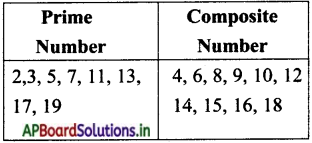Fun activity: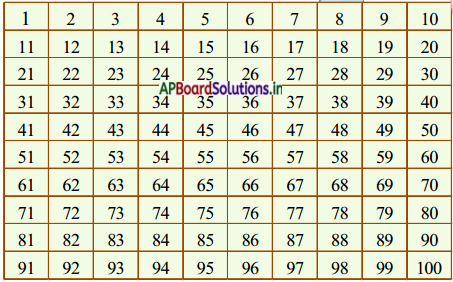Question 1.
What are the prime numbers between 1 to 10 ?
2, 3, 5, 7.

Question 2.
What are the prime numbers between 10 to 20 ?
11, 13, 17, 19.Question 3.
What are the prime numbers between 20 to 50 ?
23, 29, 31, 37, 41, 43, 47.

Question 4.
How many prime numbers are there between 1 to 50 ?
15 prime numbers are there. (2, 3, 5, 7, 11, 13, 17, 19, 23, 29, 31, 37, 41, 43, 49).

Question 5.
What are the prime numbers between 50 to 100 ?
53, 59, 61, 67, 71, 73, 79, 83, 87, 89, 97.

Question 6.
How many prime numbers are there between 50 to 100 ?
10 prime numbers are there between 50 to 100.

Question 7.
Do you observe any speciality in these prime numbers ? What is it ?
All numbers are odd, ‘2’ is only one even prime.

Question 8.
Are the all prime numbers even or odd ?
All are odd except 2.Do these: (TextBook Page No.78)

a) 52
b) 100
c) 88
d) 96
e) 90
a)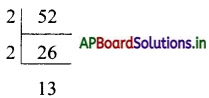∴ Prime factorisation of 52 = 2 × 2 × 13

b)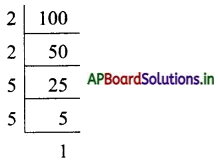∴ Prime factorisation of 100 = 2 × 2 × 5 × 5

c)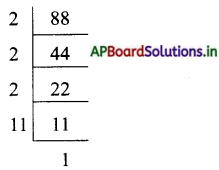∴ Prime factorisation of 88 = 2 × 2 × 2 × 11d)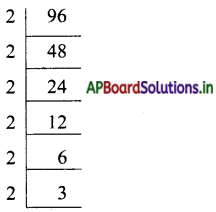∴ Prime factorisation of 96 = 2 × 2 × 2 × 2 × 2 × 3

e)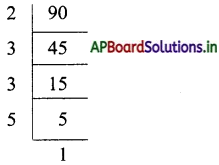∴ Prime factorisation of 90 = 2 × 3 × 3 × 5

Question 2.
The prime factorisation of 12 × 15 is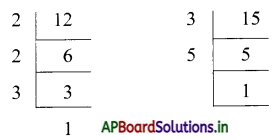∴ 12 × 15 = 2 × 2 × 3 × 3 × 5.Question 3.
Match the following: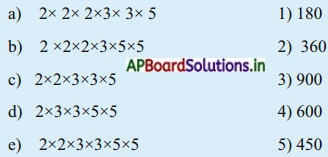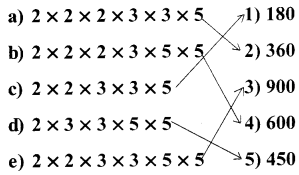Question 4.
5 × 2 × 3 × 3 is the prime factorisation of _________
90

Do this: (TextBook Page No.79)

Question 1.
Find the common factors of the following numbers and represent in the diagram.
a) 6 and 12
b) 12 and 20
c) 9 and 18
d) 11 and 22
a) Factors of 6 = 1, 2, 3, 6
Factors of 12 = 1, 2, 3, 4, 6, 12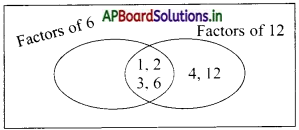b) Factors of 12 = 1, 2, 3, 4, 6, 12
Factors of 20 = 1, 2, 4, 5, 10, 20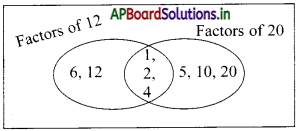c) Factors of 9 = 1, 3, 9
Factors of 18 = 1, 2, 3, 6, 9, 18d) Factors of 11 = 1, 11
Factors of 22 = 1,2, 11, 22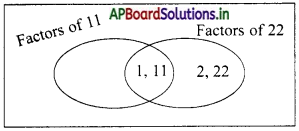Do this: (TextBook Page No.80)

Find the HCF of the following pairs of numbers by writing common factors.
1) 21 and 28
2) 34 and 20
3) 33 and 39
4) 16 and 36
5) 12 and 18
6) 80 and loo
1) Factors of 21 = 1, 3, 7, 21
Factors of 28 = 1, 2, 4, 7, 14, 28
Common factors of 21 and 28 = 1, 7
HCF of 21 and 28 = 7.

2) Factors of 34 = 1, 2, 17, 34
Factors of 20 = 1, 2, 4, 5, 10, 20
Common factors of 34 and 20 = 1, 2
HCF of 34 and 20 = 1, 2.

3) Factors of 33 = 1, 3, 11, 33
Factors of 39 = 1, 3, 13, 39
Common factors of 33 and 39 = 1, 3
HCF of 33 and 39 = 3.

4) Factors of 16 = 1, 2, 4, 8, 16
Factors of 36 = 1, 2, 3, 4, 9, 12, 18, 36
Common factors of 16 and 36 = 1, 2, 4

5) Factors of 12 = 1, 2, 3, 4, 6, 12
Factors of 18 = 1, 2, 3, 6, 9, 18
Common factors of 12 and 18 = 1,2, 3, 6
HCF of 12 and 18 = 6

6) Factors of 80 = 1, 2, 4, 5, 8, 10, 16, 20, 40, 80
Factors of 100 = 1, 2, 4, 5, 10, 20, 25, 50, 100
Common factors of 80 and 100 = 1, 2, 4, 5, 10, 20
HCF of 80 and 100 = 20.Try this: (TextBook Page No.80)

Find the HCF for the following pair of numbers. What do you observe ?
1) 4, 16
2) 4, 12
3) 5, 15
4) 14, 42
1) Factors of 4 = 1, 2, 4
Factors of 16 = 1,2, 4, 8, 16
Common factors of 4 and 16 = 1, 2, 4
HCF of 4 and 16 = 4.

2) Factors of 4 = 1, 2, 4
Factors of 12 = 1,2, 3, 4, 6, 12
Common factors of 4 and 12 = 1, 2, 4
HCF of 4 and 12 = 4.

3) Factors of 5 = 1, 5
Factors of 15 = 1, 3, 5, 15
Common factors of 5 and 15 = 1, 5
HCF of 5 and 15 = 5.

4) Factors of 14 = 1, 2, 7
Factors of 42 = 1, 2, 3, 6, 7, 14, 21, 22
Common factors of 14 and 42 = 1, 2, 7
HCF of 14 and 42 = 7

Observation :
In pair of numbers, if one of them is multiple of the other. The smallest number is the HCF of the pair of number.Do these: (TextBook Page 82)

Question 1.
Find LCM and HCF by prime factorisation method for the following.
a) 15, 48
b) 18, 42, 48
c) 15, 25, 30
d) 10, 15, 25
e) 15, 18, 36, 20
a) Given numbers 15 and 48.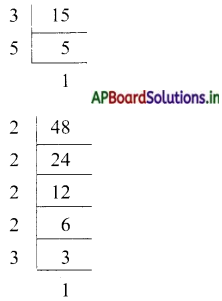Prime factorisation of 15 = 1 × 3 × 5
Prime factorisation of 48 = 2 × 2 × 2 × 2 × 3
Common factors = 1 × 3
Other factors = 5 × 2 × 2 × 2 × 2
LCM = 1 × 3 × 5 × 2 × 2 × 2 × 2 = 240
HCF = 1 × 3 = 3.b) Given numbers 18, 42 and 48.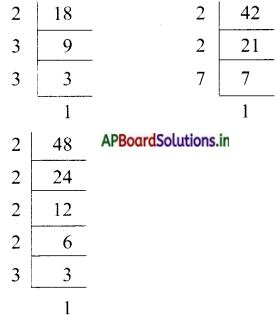Prime factorisation of 18 = 1 × 2 × 3 × 3
Prime factorisation of 42 = 1 × 2 × 3 × 7
Common factors = 1 × 2 × 3
Other factors = 3 × 7 × 2 × 2 × 2
LCM = 1 × 2 × 3 × 3 × 7 × 2 × 2 × 2 = 1008
HCF = 1 × 2 × 3 = 6

c) Given numbers 15, 25 and 30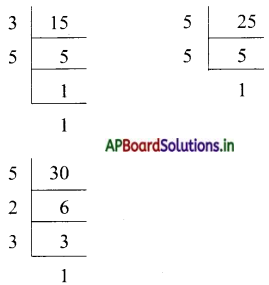Prime factorisation of 15 = 1 × 3 × 5
Prime factorisation of 25 = 1 × 5 × 5
Prime factorisation of 30 = 1 × 2 × 3 × 5
Common factors = 1 × 5
Other factors = 3 × 3 × 5 × 2
LCM = 1 × 5 × 3 × 3 × 5 × 2 = 450
HCF = 1 × 5 = 5.d) Given numbers 10, 15 and 25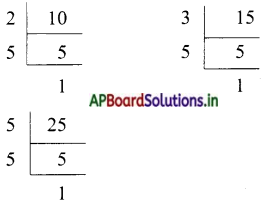Prime factorisation of 10 = 1 × 2 × 5
Prime factorisation of 15 = 1 × 3 × 5
Prime factorisation of 25 = 1 × 5 × 5
Common factors = 1 × 5 = 5
Other factors = 2 × 3 × 5 = 30
LCM = 5 × 30 = 150
HCF = 5

e) Given numbers 15, 18, 36 and 20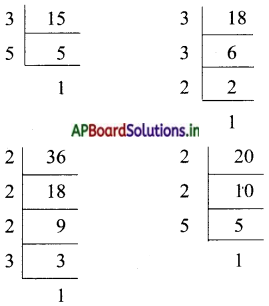Prime factorisation of 15 = 1 × 3 × 5
Prime factorisation of 18 = 1 × 2 × 3 × 3
Prime factorisation of 36 = 1 × 3 × 3 × 2 × 2
Prime factorisation of 20 = 1 × 2 × 2 × 5
Common factors = 1
Other factors = 3 × 5 × 2 × 3 × 3 × 3 × 2 × 2 × 2 × 5 = 194,400
LCM = 194, 400
HCF = 1.Question 2.
Find LCM and HCF by division method.
a) 16, 28, 36
b) 12, 18, 42
c) 30, 75, 90
d) 24, 32, 48
e) 12, 15, 18

a) Given numbers 16, 28 and 36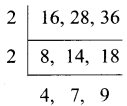LCM = 2 × 2 × 4 × 7 × 9 = 1008
HCF = 2 × 2 = 4

b) Given numbers 12, 18 and 42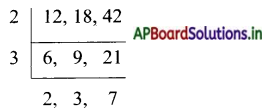LCM = 2 × 3 × 2 × 3 × 7 = 252
HCF = 2 × 3 = 6

c) Given numbers 30, 75, 90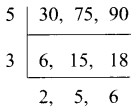LCM = 5 × 3 × 2 × 5 x 6
HCF = 5 × 3 = 15

d) Given numbers 24, 32, 48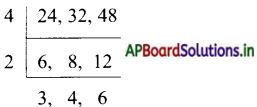LCM = 4 × 2 × 3 × 4 × 6 = 576
HCF = 4 × 2 = 8

e) Given numbers 12, 15 and 18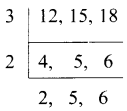LCM = 3 × 2 × 2 × 5 × 6 = 360
HCF = 3Exercise 3:

Solve the following word-problems:

Question 1.
There are some fruits in a basket. If we arrange 4 or 6 or 8 or 10 fruits in a pile, no fruits are left in the bas¬ket. What is the minimum number of fruits in the basket ?Arrangement of fruits in a pile are 4 or 6 or 8 or 10
We need to find the LCM of 4, 6, 8, 10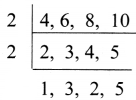LCM = 2 × 2 × 1 × 3 × 2 × 5 = 120
The minimum number of fruits in the basket = 120.

Question 2.
Ramu has 16 blue marbles and 12 white ones. If he wants to arrange them in identical groups without leaving any marbles, what is the maximum number in each group Ramu can make?Number of marbles Ramu has = 16 and 12
We need to find the HCF of 12 and 16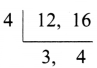HCF = 4
Maximum number of marbles Ramu can make = 4.Question 3.
Two Neon lights are turned on at the same time. One blinks for every 4 seconds and other blinks for every 6 seconds. In 60 seconds how many times will they blink at a time ?Blink time of lights = 4 sec and 6 sec
LCM of 4 and 6 =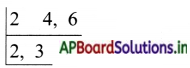LCM = 2 × 2 × 3 = 12 sec
The lights blinks at a time for every 12 sec.
Given time = 60 sec
Number of times will the lights blink = 60 ÷ 12 = 5 times.

Question 4.
There are 40 girls and 32 boys, who want to participate in state-level games competition. If each team must have the same number of girls and the same number of boys.
i) What is the maximum number in each team that can participate in state-level games ?
ii) How many boys and girls will be on each team?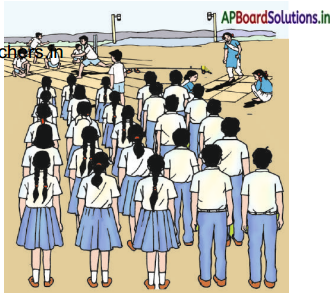Number of boys 32 and girls participate in the game is 32 and 40
i) To find maximum number in each team can participate in state-level game, we need to find
HCF of 32 and 40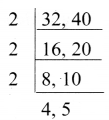∴ HCF = 2 × 2 × 2 = 8
The maximum number in each team = 8 numbers.

ii) To find out boys and girls will be an each team, we find LCM of 32 and 40.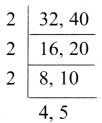∴ LCM of 32 and 40 = 2 × 2 × 2 × 4 × 5 = 160.

Question 5.
Find the least number of sheets of paper required to make notebooks containing 32 sheets or 40 sheets or 48 sheets without a single sheet leaving behind.To find the least number of sheets of paper required to make note books, we need to find LCM of 32, 40, 48.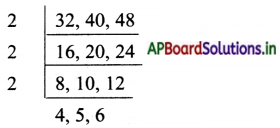LCM = 2 × 2 × 2 × 4 × 5 × 6 = 960
∴ 960 of sheets of paper required to make notebooks containing given sheets.Question 6.
What is the least number of chairs needed for an auditorium so that they can be arranged either 27 in a row or 33 in a row?